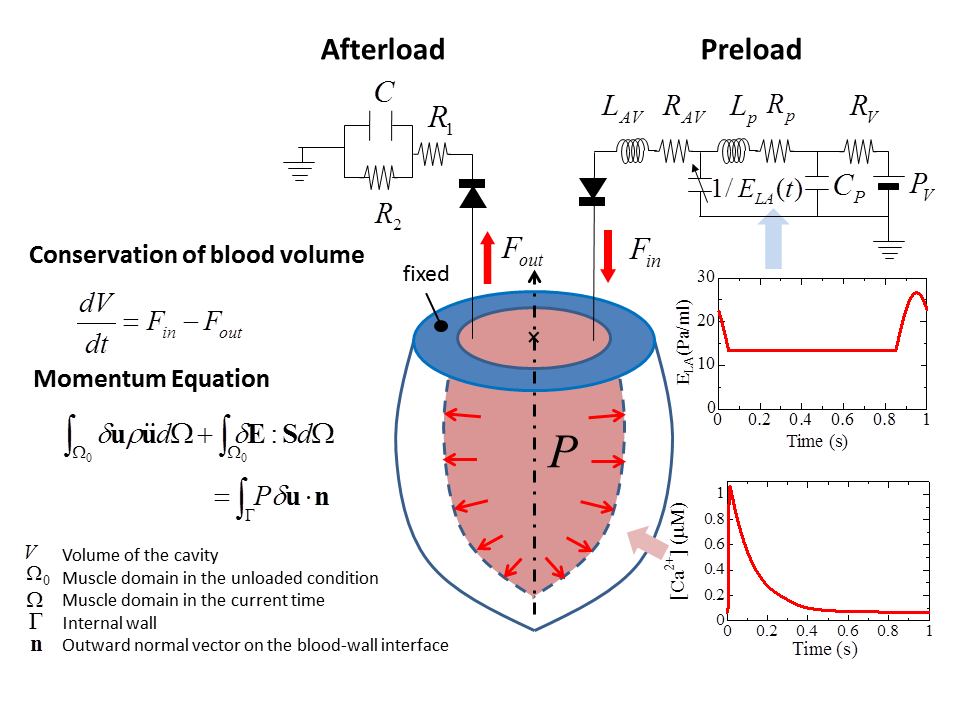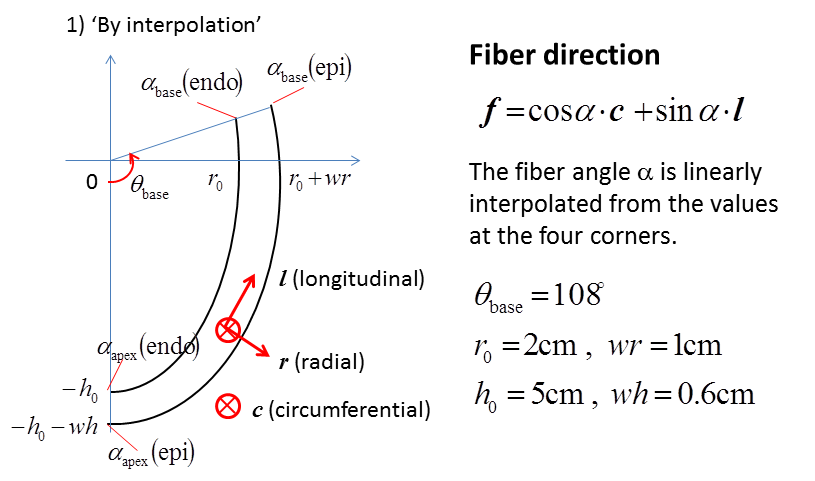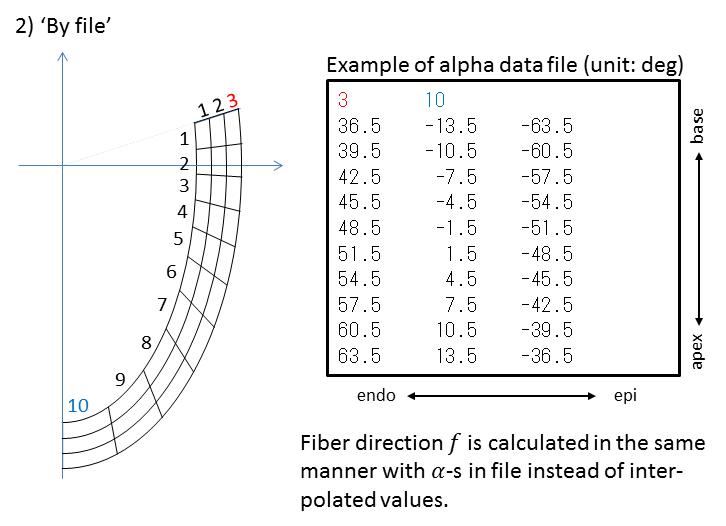## Heart Beat

In this test, the beating heart simulation is performed with a rotationally symmetric left ventricle model. The same time profile of calcium ion concentration as in Isometric Contraction is given for all places in the muscle. The half cross sectional area is divided into quadratic elements, and your sarcomere model is imbedded into each of elements. The momentum equation of the heart wall is solved under the equilibrium condition with the intracavity pressure P, where the equilibrium conditions with the circuit models are also imposed. The basal portion of the model is fixed in the simulation.The geometry in the unloaded condition of the half sectional model is given by ellipsoidal curves. At each point in the heart wall model, the normal frame composed of so called the cardiac vectors c, l and r is given. The longitudinal unit vector l is a vector directed from the apex to the base and parallel to the ellipsoidal curve. The circumferential unit vector c is a vector perpendicular to the sectional plane and directed to the back of the plane. The radial vector is given by r=c×l. In the heart beat test, we assume that the fiber orientation f is perpendicular to the radial direction r and given by the rotational angle α from the circumferential vector c. You can specify the angles α in the following ways:

1. by using linear interpolation of the four angles on the corners ('by interpolation')
2. from data file you uploaded to the server ('by file')
before submitting your job through the Preferences in the Simulation Setup Dialog.